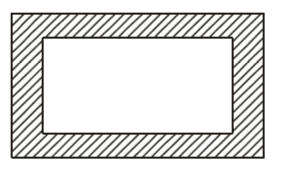d A path of uniform width runs round the inside jemrare.com

# Area Calculation MCQ 3 (A path of uniform width runs round the inside)

Area Calculation MCQ 3: A path of uniform width runs round the inside of a rectangular field 38 m long and 32 m wide. if the path occupies 600m2, then the width of the path is_______?

a) 3 m                   b) 5 m                      c) 8 m                   d) 10 m

Solution:We know that;

$Area of rectangle = Length \times Width$As per given length and width the are of the rectangle is ;

$$Area of The rectangle = 38\times32=1216 m^2$$

From this area we subtract the area of the path;

==>$Area Of Small Rectangle Surrounded By The Path=1216-600=616 m^2$

i-e for inner rectangle ​$$Length \times Width = 616$$

But what is the length and width of inner triangle ?

To find this we suppose that the path has width ‘x’

Then from fig we can see that

Length of smaller rectangle = (38-2x) m

Width of inner rectangle = (32-2x) m

So now we can write ;

==> $(38-2x)(32-2x)=616$

== >$1216-76x-64x+4x^2=616$

which gives $4x^2-140x+600=0$

this gives us $x^2-35x+150=0$

we are there $x^2-30x-5x+150=0$

$==> (x-30)(x-5)=0$

==> x= 30 or x=5

If we choose x = 30  as our answer then as we set width of smaller rectangle to be (38-2x) – will become 38-2(30)=38-60=-22 which is wrong – because a length is never -ve !

So we choose x=5 m as our answer.

So option b is correct!Copyright secured by Digiprove © 2020-2021